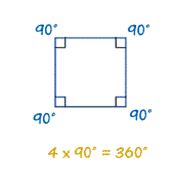Problems
10037 - Angles of Polygon

Angles of Polygon

Time Limit: 1 sec

The Problem

We know that, sum of all the angles in a triangle is 180 degree and sum of all the angles in a rectangle is 360 degree.Now, what is the sum of all the angles of a polygon which has N sides?

The Input

Input contains a single integer N (3≤ N ≤106) per line till the end of file(EOF).

The Output

Print a single integer for every line of input, the sum of all the angles of a polygon which has N sides in a separate line.

Sample Input

3

4

Sample Output

180

360

[College Level]

1st Online Programming Contest 2016 by Outsbook

Problem Setter: Fahim Abrar